# In and out

The empty tank is filled in 12 minutes and empty in 16 minutes. How long does it take to fill if we forgot to close the drain?
If the tank has 1000 l.

t =  48 min

### Step-by-step explanation:

t•(1/12 - 1/16)=1

t = 48

Our simple equation calculator calculates it.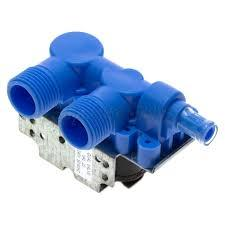Did you find an error or inaccuracy? Feel free to write us. Thank you!Tips to related online calculators
Looking for calculator of harmonic mean?
Looking for a statistical calculator?
Do you have a linear equation or system of equations and looking for its solution? Or do you have a quadratic equation?
Do you want to convert time units like minutes to seconds?

## Related math problems and questions:

• Fire tank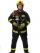Whole fire tank was discharged once in 5 days by first out by second in \$n days. Once firefighters pumped out 6/13 of water out from the tank and then let the water flow out both drain. How long take empty the tank?
• Two pumpsPump A takes twice as long to fill a tank as B. Together they take 2 hours to fill the tank. How long does each one take to fill the tank by itself?
• Inflow - outflow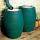The tank will fill by inflow for 143 minutes and empties by outflow for 150 minutes. How long take to fill tank if it is also opened the inflow and outflow?
• Two pumps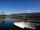The first pump fills the tank in 24 hours second pump in another 40 hours. For how long will it take to fill the tank if it operates both pumps at the same time?
• Water reservoir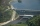One drain hole emptied the water from the tank for 20 hours, with the second drain for 30 hours. How long will the water drain from this tank if we open two drains simultaneously?
• Oil tank and pipes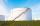The underground oil tank can be filled by two oil pipelines. The first is filled in 72 hours and the second in 48 hours. How many hours from the moment when first pipeline began to fill the oil is it necessary to start filling it with the second to fill i
• Three pumpsWe are filling the pool. The first pump would be filled in 12 hours, the second pump in 15 hours. If all three pumps were running at the same time, it would fill the pool for 4 hours. How long would the pool fill only with the third pump?
• PumpingThe tank is filled with a larger pump in 12 hours and with scond pump in 15 hours. For how long does the tank fill when both pumps are switched on at the same time?
• Water inlets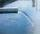Inlet valve with a flow rate of 12 liters per second is filled tank for 72 minutes. How long take to fill full tank if we open one more such valve half an hour after?
• Five inlets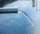The tank can be filled with five equally powerful inlets. If the tank is filled by four of these inlets, it takes a total of 30 minutes to fill one-third of the tank. How many minutes does it take to fill an empty tank if it is filled with all five inlets
• Tank No 8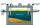Tank is filled by one inlet valve with a flow rate of 12 liters per second in 72 minutes. How long take the tank to fill, if we open half an hour after one more inlet?
• Pumps A and BPump A fill the tank for 12 minutes, pump B for 24 minutes. How long will take to fill the tank if he is only three minutes works A and then both pumps A and B?
• Two rooms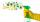Woman cleans the room in 30 minutes a man in 90 minutes. How long will take they clean two rooms?
• Water reservoirThe water reservoir is filled with one pump for 4 days by the second one for 9 days. Outlet can drain tank in 12 days. How long take fill the reservoir if both pumps are running and not closed outlet channel.
• Mow the lawn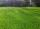Dano would mow the lawn in 12 hours and Milada in 16 hours. How long will take the lawn mow together?
• Collection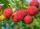John has collected half a bucket of raspberries in 45 minutes, Eva collects the whole bucket in an hour. How many minutes it would take to fill a bucket if the two children work together?
• Tributaries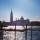The first tributary fill pool with water in 15 hours. The second tributary fill pool in 10 hours. For how many hours the pool is filled with both tributaries?